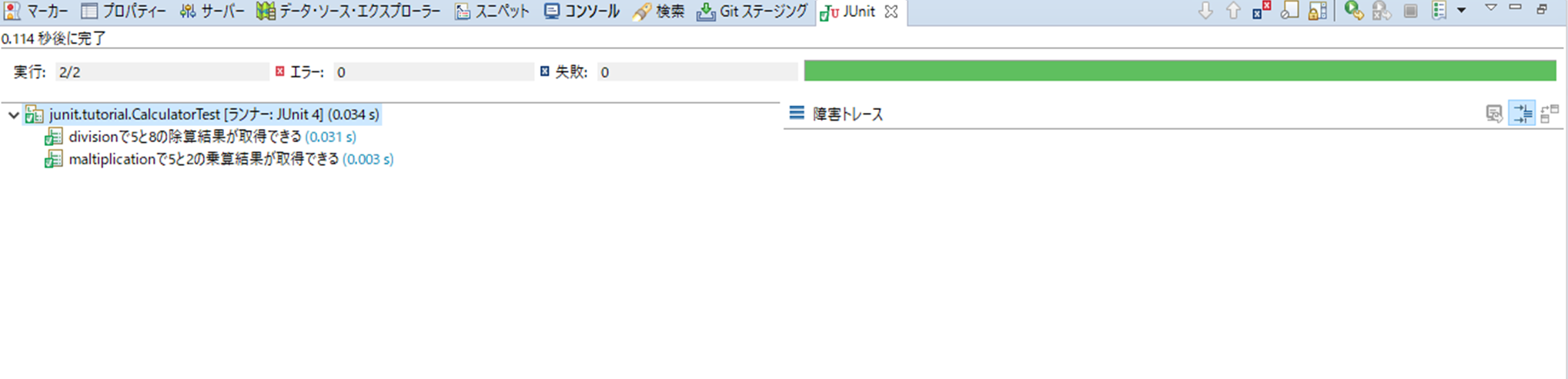# environment

・ Windows10 ・ Jdk 1.8.0_201 -Eclipse Version: 2018-12 (4.10.0) ・ Junit_4.12.0

# Target

Being able to run unit tests for classes created in java.

# What is Junit

Java version of xUnit testing framework for created classes You can test the operation by calling the method of the class under test from the created Junit code.

Since the test code created once can be reused, compared to the method of creating test code every time The man-hours required for testing can be reduced.

# Precautions for testing with Junit

1. Must be a public method.
2. The return value is void.
3. It has no arguments.
4. Annotate the test method with org.junit.Test.

# Create a class to be tested.

This time, I created a class Calculator.java that performs multiplication and division.

#### `Calculator.java`

``````
package junit.tutorial;

public class Calculator {

/**
*Returns the result of multiplying x and y
* @param x
* @param y
* @return Returns the result of multiplying x and y
*/
public int maltiplication(int x,int y) {
return x * y;
}

/**
*Returns the division result of x and y
* @param x
* @param y
* @return Returns the division result of x and y
*/
public int division(int x,int y) {
return x / y;
}
}
``````

# Create test code.

Next, create a test class. If you create a new class, enter test and then ctrl + space Eclipse Content Assist will start, so select the template for Junit 4 You can easily create a method.

First, create a test class CalculatorTest class with nothing in it.

#### `CalculatorTest.java`

``````
package junit.tutorial;

public class CalculatorTest {
}

``````

Insert the template method here using the above content assist.

#### `CalculatorTest.java`

``````
package junit.tutorial;

import static org.junit.Assert.*;

import org.junit.Test;

public class CalculatorTest {
@Test
public void testName() throws Exception {

}
}

``````

# Describe in the test code.

This time, we will create the test code as follows. Finally, by confirming the result of 3, it can be judged whether the test worked as expected.

1. Set the input value used in the method.
2. Set the expected value of the return value of the method.
3. Assert the expected value and the measured value, which is the return value of 1.

# Complete the test code.

Complete the test code immediately. The method name is Japanese. This allows you to see at a glance what the execution result has verified.

#### `Calculator.java`

``````
package junit.tutorial;

import static org.hamcrest.CoreMatchers.*;
import static org.junit.Assert.*;

import org.junit.Test;

public class CalculatorTest {

@Test
You can get the multiplication result of 5 and 2 with public void maltiplication() throws Exception {
Calculator sut = new Calculator();
int expected = 10;
int actual = sut.maltiplication(5, 2);
assertThat(actual,is(expected));
}

@Test
You can get the division result of 5 and 8 by public void division() throws Exception {
Calculator sut = new Calculator();
int expected =

}
}

``````

While setting the expected value of the test code, I notice that the return value is not good as a result of division if it is int. In response to this fact, the class under test is modified.

#### `Calculator.java`

``````
package junit.tutorial;

public class Calculator {

/**
*Returns the result of multiplying x and y
* @param x
* @param y
* @return Returns the result of multiplying x and y
*/
public int maltiplication(int x,int y) {
return x * y;
}

/**
*Returns the division result of x and y
* @param x
* @param y
* @return Returns the division result of x and y
*/
public float division(int x,int y) {
return (float)x / (float)y;
}
}
``````

I set the return type to float and cast the return value. Now back to test code creation.

#### `CalculatorTest.java`

``````
package junit.tutorial;

import static org.hamcrest.CoreMatchers.*;
import static org.junit.Assert.*;

import org.junit.Test;

public class CalculatorTest {

@Test
You can get the multiplication result of 5 and 2 with public void maltiplication() throws Exception {
Calculator sut = new Calculator();
int expected = 10;
int actual = sut.maltiplication(5, 2);
assertThat(actual,is(expected));
}

@Test
You can get the division result of 5 and 8 by public void division() throws Exception {
Calculator sut = new Calculator();
float expected = 0.625f;
float actual =  sut.division(5, 8);
assertThat(actual,is(expected));

}
}

``````

You can now run the test. Now, let's run a test as Junit in Eclipse and check the result.-All indicators are green on the screen. -The execution result must be completed in 2/2.

From the above results, it was found that the method worked as expected.

This is the basic operation of Junit.

I would also like to study the outline of the exam using Junit.

# References

[Introduction to JUnit practice ── Unit test technique to learn systematically WEB + DB PRESS plus] https://www.amazon.co.jp/dp/B07JL78S95/ref=dp-kindle-redirect?_encoding=UTF8&btkr=1